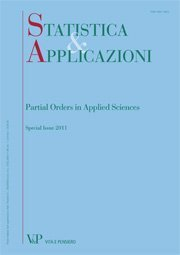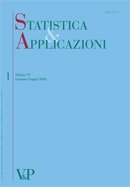Libri di Raffaella Calabrese - libri Statistica & Applicazioni

# Raffaella Calabrese

### Author's titles

Regression model for proportions with probability masses at zero and one digitalYear: 2012
SUMMARY In many settings, the variable of interest is a proportion with high concentration of data at the boundaries. This paper proposes a regression model for a fractional variable with nontrivial probability masses at the extremes. In particular, the dependent variable is assumed to be a mixed random variable, obtained as the mixture of a Bernoulli and a beta random variables. The endpoints of zero and one are modelled by a logistic regression model. The values belonging to the interval (0,1) are assumed to be beta distributed and their mean and dispersion are jointly modelled by using two link functions. The regression model proposed here accommodates skewness and heteroscedastic errors. Finally, an application to loan recovery process of Italian banks is also provided. Keywords: Proportions, Mixed Random Variable, Beta Regression, Skewness, Heteroscedasticity.
Measuring loan recovery rate: methodology and empirical evidence digitalYear: 2008
This paper aims at proposing a new methodology to compute recovery rate on non-performing bank loans, in order to confine this variable within the interval [0,1]. Such a methodology is then applied to data on loans gathered by the Bank of Italy and some interesting characteristics of the loan recovery process in the Italian banking market are highlighted. The combined effects of some variables on the recovery rates are also analysed. In particular, the presence of either collateral or personal guarantee, the borrower’s residence area are considered, thereby emphasizing the relationship between the recovery rate and the total exposure.